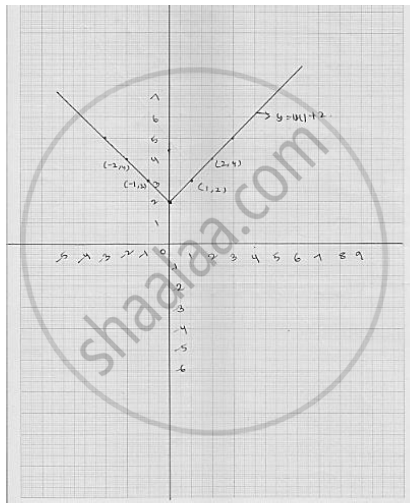# Draw the Graph of Y = | X | + 2. - Mathematics

Draw the graph of y = | x | + 2.

#### Solution

We have

y = | x | + 2          ............. (1)

Putting   x = 0 , we get y  = 2 ..............

Putting   x = 1 , we get y = 3

Putting   x  = - 1 , we get  y = 3

Thus, the we have the following table for the points on graph of  | x | + 2

 x 0 1 1 y 2 3 3

Graph of line equation   y = | x | + 2Concept: Graph of a Linear Equation in Two Variables
Is there an error in this question or solution?

#### APPEARS IN

RD Sharma Mathematics for Class 9
Chapter 7 Linear Equations in Two Variables
Exercise 7.3 | Q 16

Share